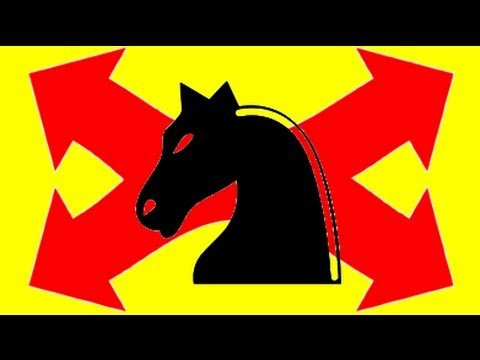# Can you Solve the Knight’s Tour Math Problem?

Solving the “Knight’s Tour” math problem involves moving the knight about the chessboard such that each square is visited exactly once. Visualizing the chessboard as 4 quadrants, memorizing a small group of patterns within each quadrant, and following a few simple principles while calculating the knight moves will allow you to find a solution to this fun mathematical problem. It’s an intuitive puzzle to challenge a friend, math teacher, or even a math classroom with.